## Monday, June 10, 2019

### SPX Iron Condor - 2019 Q1 Review

We looked at the performance of a few of the better performing SPX iron condors (ICs) in a prior article (here). In this post, we'll revisit those IC variations and see how they performed in the first quarter of this year. Their performance will be compared to their historical performance from January 2007 through December 2018.

These are the IC variations we will review:
1. 66 DTE - 25 pt wings, 12 Delta (200:50) / 2 DTE - exit if the trade has a loss of 200% of its initial credit OR if the trade has a profit of 50% of its initial credit OR at 2 DTE.
2. 66 DTE - 25 pt wings, 12 Delta (200:50) / 33 DTE - exit if the trade has a loss of 200% of its initial credit OR if the trade has a profit of 50% of its initial credit OR at 33 DTE.
3. 73 DTE - 25 pt wings, 12 Delta (200:50) / 2 DTE - exit if the trade has a loss of 200% of its initial credit OR if the trade has a profit of 50% of its initial credit OR at 2 DTE.
4. 73 DTE - 25 pt wings, 12 Delta (200:50) / 37 DTE - exit if the trade has a loss of 200% of its initial credit OR if the trade has a profit of 50% of its initial credit OR at 37 DTE.
5. 45 DTE - 25 pt wings, 16 Delta (200:50) / 2 DTE - exit if the trade has a loss of 200% of its initial credit OR if the trade has a profit of 50% of its initial credit OR at 2 DTE.
6. 45 DTE - 25 pt wings, 16 Delta (200:50) / 22 DTE - exit if the trade has a loss of 200% of its initial credit OR if the trade has a profit of 50% of its initial credit OR at 22 DTE.

The 45 DTE variations follow the entry and exit criteria popularized by TastyTrade here:

For each IC variation, I show one table and two charts. The table shows the percent return on reg-t margin. The first chart shows these same return numbers, but compared to their historical returns (max, min, average, and quartiles).The second chart shows the DIT numbers for each variation compared to the average for this variation.

Let's get right to the results for each of these variations.

66 DTE - 25 pt wings, 12 Delta (200:50) / 2 DTE

The average monthly return for Q1 was -7%, versus the 2007 to 2018 monthly average of +3%. Total return for the quarter was -21%. Pretty bad return numbers. The average DIT for Q1 was 34, which was above the 2007 to 2018 average of 30 DIT.(click to enlarge)(click to enlarge)(click to enlarge)

66 DTE - 25 pt wings, 12 Delta (200:50) / 33 DTE

The average monthly return for Q1 was +5%, versus the 2007 to 2018 monthly average of +4%. Total return for the quarter was +15%. One of the few trades/variations (ICs, Straddles, Strangles) this quarter with positive quarterly return numbers. The average DIT for Q1 was 29, which was above the 2007 to 2018 average of 25 DIT.(click to enlarge)(click to enlarge)(click to enlarge)

73 DTE - 25 pt wings, 12 Delta (200:50) / 2 DTE

The average monthly return for Q1 was -10%, versus the 2007 to 2018 monthly average of +2%. Total return for the quarter was -29%. Pretty bad return numbers. The average DIT for Q1 was 36, which was above the 2007 to 2018 average of 33 DIT.(click to enlarge)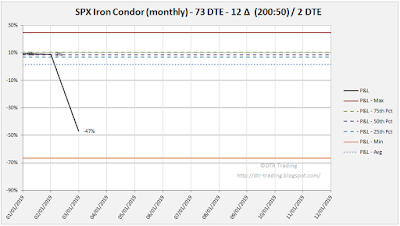(click to enlarge)(click to enlarge)

73 DTE - 25 pt wings, 12 Delta (200:50) / 37 DTE

The average monthly return for Q1 was -3%, versus the 2007 to 2018 monthly average of +1%. Total return for the quarter was -9%. Not great return numbers, but still tradeable.  The average DIT for Q1 was 33, which was above the 2007 to 2018 average of 28 DIT.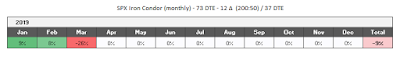(click to enlarge)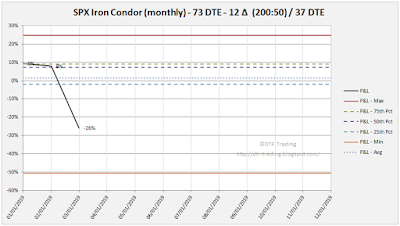(click to enlarge)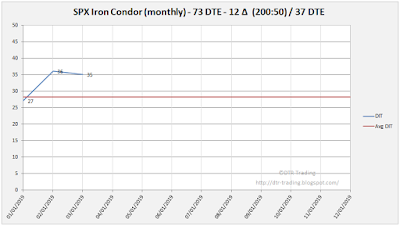(click to enlarge)

45 DTE - 25 pt wings, 16 Delta (200:50) / 2 DTE

The average monthly return for Q1 was -34%, versus the 2007 to 2018 monthly average of +3%. Total return for the quarter was -101%! Horrible return numbers for this IC variation! The average DIT for Q1 was 37, which was above the 2007 to 2018 average of 24 DIT.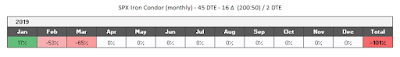(click to enlarge)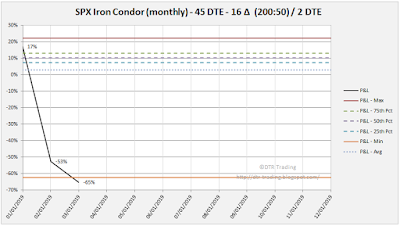(click to enlarge)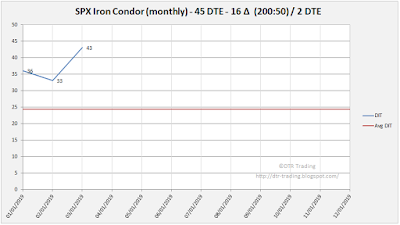(click to enlarge)

45 DTE - 25 pt wings, 16 Delta (200:50) / 22 DTE

The average monthly return for Q1 was -18%, versus the 2007 to 2018 monthly average of +2%. Total return for the quarter was -53%. Pretty lousy returns for Q1. The average DIT for Q1 was 23, which was above the 2007 to 2018 average of 20 DIT.(click to enlarge)(click to enlarge)(click to enlarge)

The 45 DTE variations were clearly the worst for the quarter. The 66 and 73 DTE variations that managed early were decent performers.

## Monday, May 27, 2019

### SPX Straddle - 2019 Q1 Review

We looked at the performance of a few of the better performing SPX straddles in a prior article (here). In this post, we'll revisit those straddle variations and see how they performed in the first quarter of this year. Their performance will be compared to their historical performance from January 2007 through December 2018.

These are the variations we will review:
1. 59 DTE - (25:10) / 2 DTE - exit if the trade has a loss of 25% of its initial credit OR if the trade has a profit of 10% of its initial credit OR at 2 DTE.
2. 59 DTE - (25:10) / 41 DTE - exit if the trade has a loss of 25% of its initial credit OR if the trade has a profit of 10% of its initial credit OR at 41 DTE.
3. 59 DTE - (50:25) / 2 DTE - exit if the trade has a loss of 50% of its initial credit OR if the trade has a profit of 25% of its initial credit OR at 2 DTE.
4. 59 DTE - (50:25) / 24 DTE - exit if the trade has a loss of 50% of its initial credit OR if the trade has a profit of 25% of its initial credit OR at 24 DTE.
5. 45 DTE - (150:25) / 2 DTE - exit if the trade has a loss of 150% of its initial credit OR if the trade has a profit of 25% of its initial credit OR at 2 DTE.
6. 45 DTE - (150:25) / 31 DTE - exit if the trade has a loss of 150% of its initial credit OR if the trade has a profit of 25% of its initial credit OR at 31 DTE.

The 45 DTE variations follow the entry and exit criteria popularized by TastyTrade here:

For each variation, I show one table and two charts. The table shows the percent return on portfolio margin. The first chart shows these same return numbers, but compared to their historical returns (max, min, average, and quartiles).The second chart shows the DIT numbers for each variation compared to the average for this variation.

Let's get right to the results for each of these variations.

59 DTE - (25:10) / 2 DTE

The average monthly return for Q1 was -21%, versus the 2007 to 2018 monthly average of +2%. Total return for the quarter was -62%. Pretty bad return numbers. The average DIT for Q1 was 14, which was below the 2007 to 2018 average of 16 DIT.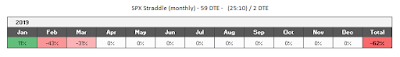(click to enlarge)(click to enlarge)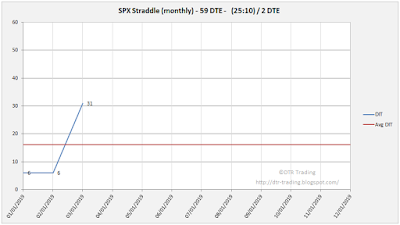(click to enlarge)

59 DTE - (25:10) / 41 DTE

The average monthly return for Q1 was -13%, versus the 2007 to 2018 monthly average of +1%. Total return for the quarter was -38%. Again, pretty bad return numbers. The average DIT for Q1 was 10, which was below the 2007 to 2018 average of 12 DIT.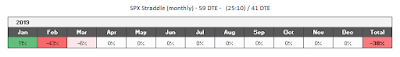(click to enlarge)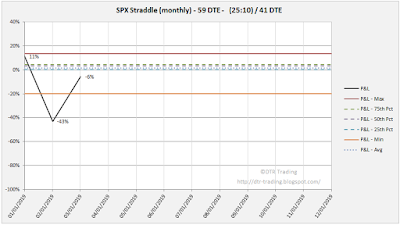(click to enlarge)(click to enlarge)

59 DTE - (50:25) / 2 DTE

The average monthly return for Q1 was -34%, versus the 2007 to 2018 monthly average of +4%. Total return for the quarter was -101%. Bad return numbers again. The average DIT for Q1 was 33, which was below the 2007 to 2018 average of 34 DIT.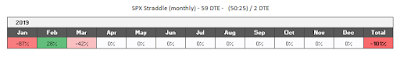(click to enlarge)(click to enlarge)(click to enlarge)

59 DTE - (50:25) / 24 DTE

The average monthly return for Q1 was -30%, versus the 2007 to 2018 monthly average of +4%. Total return for the quarter was -89%. The bad return numbers continue! The average DIT for Q1 was 30, which was above the 2007 to 2018 average of 29 DIT.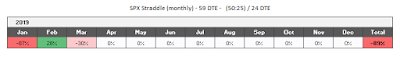(click to enlarge)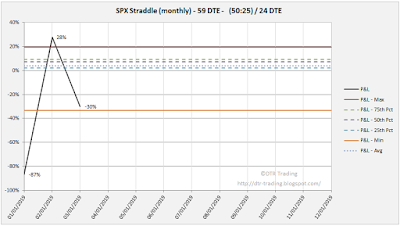(click to enlarge)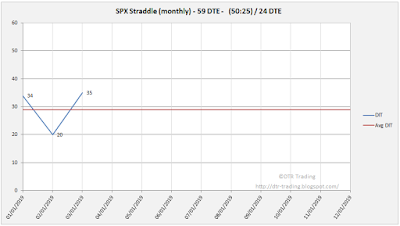(click to enlarge)

45 DTE - (150:25) / 2 DTE

The average monthly return for Q1 was -78%, versus the 2007 to 2018 monthly average of +2%. Total return for the quarter was -234%! Horrible return numbers for the quarter! The average DIT for Q1 was 35, which was above the 2007 to 2018 average of 28 DIT.(click to enlarge)(click to enlarge)(click to enlarge)

45 DTE - (150:25) / 31 DTE

The average monthly return for Q1 was -11%, versus the 2007 to 2018 monthly average of +1%. Total return for the quarter was -33%. Pretty lousy returns for the Q1. The average DIT for Q1 was 14, which was the same as the 2007 to 2018 average DIT.(click to enlarge)(click to enlarge)(click to enlarge)

All of the straddle variations had negative returns for the quarter. In the next post, we'll review the Q1 returns for the SPX Iron Condor variations.

## Monday, May 20, 2019

### SPX Strangle - 2019 Q1 Review

We looked at the performance of a few of the better performing SPX strangles in a prior article (here). In this post, we'll revisit those strangle variations and see how they performed in the first quarter of this year. Their performance will be compared to their historical performance from January 2007 through December 2018.

These are the variations we'll review:
1. 59 DTE - 16 Delta Short Strikes (100:50) / 2 DTE - exit if the trade has a loss of 100% of its initial credit OR if the trade has a profit of 50% of its initial credit OR at 2 DTE.
2. 59 DTE - 16 Delta Short Strikes (100:50) / 29 DTE - exit if the trade has a loss of 100% of its initial credit OR if the trade has a profit of 50% of its initial credit OR at 2 DTE.
3. 59 DTE - 16 Delta Short Strikes (200:50) / 2 DTE - exit if the trade has a loss of 200% of its initial credit OR if the trade has a profit of 50% of its initial credit OR at 2 DTE.
4. 59 DTE - 16 Delta Short Strikes (200:50) / 29 DTE - exit if the trade has a loss of 200% of its initial credit OR if the trade has a profit of 50% of its initial credit OR at 2 DTE.
5. 45 DTE - 16 Delta Short Strikes (200:50) / 2 DTE - exit if the trade has a loss of 200% of its initial credit OR if the trade has a profit of 50% of its initial credit OR at 2 DTE.
6. 45 DTE - 16 Delta Short Strikes (200:50) / 22 DTE - exit if the trade has a loss of 200% of its initial credit OR if the trade has a profit of 50% of its initial credit OR at 2 DTE.

The 45 DTE variations follow the entry and exit criteria popularized by TastyTrade here:

For each variation, I show one table and two charts. The table shows the percent return on portfolio margin. The first chart shows these same return numbers, but compared to their historical returns (max, min, average, and quartiles).The second chart shows the DIT numbers for each variation compared to the average for this variation.

Let's get right to the results for each of these variations.

59 DTE - 16 Delta Short Strikes (100:50) / 2 DTE

The average monthly return for Q1 was -12%, versus the 2007 to 2018 monthly average of +3%. Total return for the quarter was -37%. Pretty bad return numbers. The average DIT for Q1 was 23, which was below the 2007 to 2018 average of 27 DIT.(click to enlarge)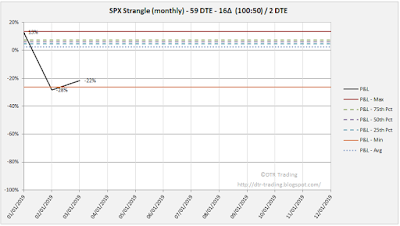(click to enlarge)(click to enlarge)

59 DTE - 16 Delta Short Strikes (100:50) / 29 DTE

The average monthly return for Q1 was -7%, versus the 2007 to 2018 monthly average of +2%. Total return for the quarter was -22%. Again, pretty bad return numbers. The average DIT for Q1 was 20, which was below the 2007 to 2018 average of 24 DIT.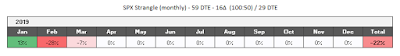(click to enlarge)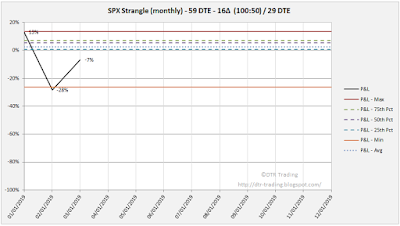(click to enlarge)(click to enlarge)

59 DTE - 16 Delta Short Strikes (200:50) / 2 DTE

The average monthly return for Q1 was +1%, versus the 2007 to 2018 monthly average of +3%. Total return for the quarter was +3%. Pretty bad return numbers, but at least we have positive returns overall. The average DIT for Q1 was 32, which was above the 2007 to 2018 average of 29 DIT.(click to enlarge)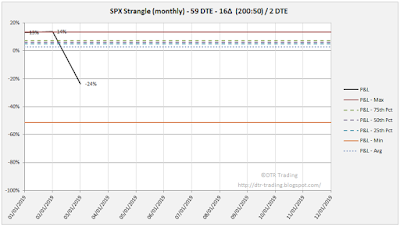(click to enlarge)(click to enlarge)

59 DTE - 16 Delta Short Strikes (200:50) / 29 DTE

The average monthly return for Q1 was +7%, versus the 2007 to 2018 monthly average of +3%. Total return for the quarter was +20%. Finally some good return numbers. The average DIT for Q1 was 23, which was below the 2007 to 2018 average of 25 DIT.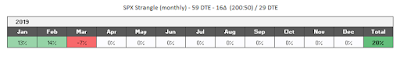(click to enlarge)(click to enlarge)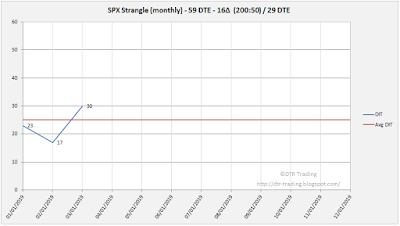(click to enlarge)

45 DTE - 16 Delta Short Strikes (200:50) / 2 DTE

The average monthly return for Q1 was -26%, versus the 2007 to 2018 monthly average of +1%. Total return for the quarter was -73%. Horrible return numbers for the quarter. The average DIT for Q1 was 32, which was above the 2007 to 2018 average of 23 DIT.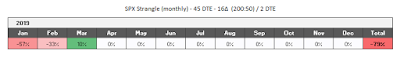(click to enlarge)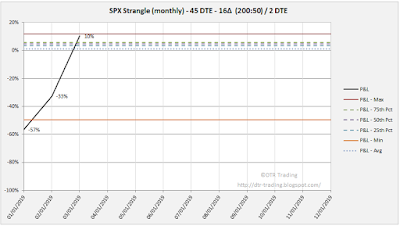(click to enlarge)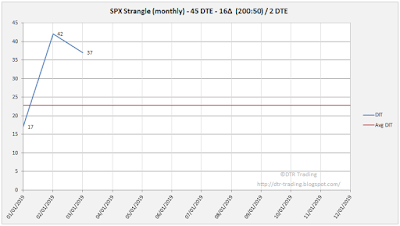(click to enlarge)

45 DTE - 16 Delta Short Strikes (200:50) / 22 DTE

The average monthly return for Q1 was -19%, versus the 2007 to 2018 monthly average of +1%. Total return for the quarter was -57%. Again, horrible return numbers for the 45 DTE variations. The average DIT for Q1 was 21, which was above the 2007 to 2018 average of 19 DIT.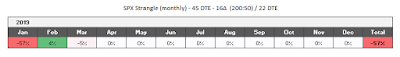(click to enlarge)(click to enlarge)(click to enlarge)

In the next post, we'll review the Q1 returns for the SPX straddle variations.